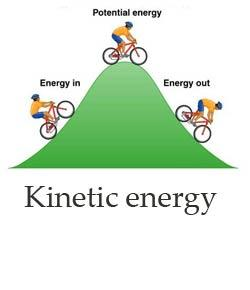# Kinetic Energy Quiz-2

5 Questions | Total Attempts: 1061SettingsChoose the correct answer from theose given

Related Topics
• 1.
The energy of an object resulting from motion is __________ energy.
• A.

Kinetic

• B.

Potential

• C.

Mechanical

• D.

Thermal

• 2.
The unit for kinetic energy is the ___
• A.

Watt

• B.

Joule

• C.

Newton

• D.

Volt

• 3.
Which of the following would produce the greatest increase in the kinetic energy of a moving object?
• A.

Doubling its mass

• B.

Doubling its velocity

• C.

Halving its mass

• D.

Halving its velocity

• 4.
A rifle can shoot a 4.20-g bullet at a speed of 965 m/s. What is the kinetic energy of the bullet as it leaves the rifle?
• A.

1.96×10^6 J

• B.

2.03 J

• C.

1.96×10^3 J

• D.

2.03×10^3 J

• 5.
A ball traveling at 30 m/s has 900 J of kinetic energy. What is the mass of the ball?
• A.

1kg

• B.

2kg

• C.

9kg

• D.

30kg